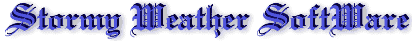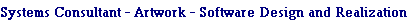Great Circle Calculator

To compute true (i.e. non-magnetic) course and distance between points, enter lat/lon of departure and arrival, select distance units and click "calculate".

Notes:

Lat/lon may be entered as:
DD.DD (degrees and decimals of a degree)
DD:MM.MM (degrees, minutes and decimals of a minute) or
DD:MM:SS.SS (degrees, minutes, seconds and decimals of a second.)
Calculations become inaccurate very close to the earth's poles due to rounding errors.

"Reciprocal" equals course at arrival plus/minus 180º.

 Input Data: Latitude departure: Longitude departure: NS WE Latitude arrival: Longitude arrival: NS WE

 Results: Initial course: Reciprocal: Distance:

Distance Units:

Earth Model: